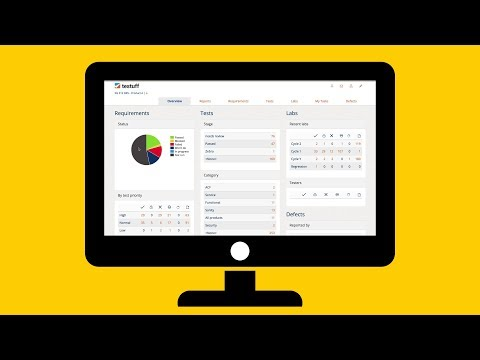``
``` /* <![CDATA[ */ jQuery(document).ready(function(\$){ \$(function(){ var width = \$(window).innerWidth(); var setwidth = 600; var ratio = 0.5; var height = 300; var link = 'https://www.youtube.com/watch?v=vSeiQvdwKms&amp;rel=0&width=600&height=300'; if(width < setwidth) { height = Math.floor(width * 0.5); //console.log("device width "+width+", set width "+600+", ratio "+0.5+", new height "+ height); var new_url = wpvl_paramReplace('width', link, width); var new_url = wpvl_paramReplace('height', new_url, height); \$("a#5fbf288d8d601").attr('href', new_url); //console.log(new_url); } }); }); /* ]]&gt; */ ```

``
``` /* <![CDATA[ */ jQuery(document).ready(function(\$){ \$(function(){ var width = \$(window).innerWidth(); var setwidth = 600; var ratio = 0.5; var height = 300; var link = 'https://www.youtube.com/watch?v=gNA9McA3kkw&amp;rel=0&width=600&height=300'; if(width < setwidth) { height = Math.floor(width * 0.5); //console.log("device width "+width+", set width "+600+", ratio "+0.5+", new height "+ height); var new_url = wpvl_paramReplace('width', link, width); var new_url = wpvl_paramReplace('height', new_url, height); \$("a#5fbf288d8d7ca").attr('href', new_url); //console.log(new_url); } }); }); /* ]]&gt; */ ```

``
``` /* <![CDATA[ */ jQuery(document).ready(function(\$){ \$(function(){ var width = \$(window).innerWidth(); var setwidth = 600; var ratio = 0.5; var height = 300; var link = 'https://www.youtube.com/watch?v=LA4UKbGgw6s&amp;rel=0&width=600&height=300'; if(width < setwidth) { height = Math.floor(width * 0.5); //console.log("device width "+width+", set width "+600+", ratio "+0.5+", new height "+ height); var new_url = wpvl_paramReplace('width', link, width); var new_url = wpvl_paramReplace('height', new_url, height); \$("a#5fbf288d8d97d").attr('href', new_url); //console.log(new_url); } }); }); /* ]]&gt; */ ```

``
``` /* <![CDATA[ */ jQuery(document).ready(function(\$){ \$(function(){ var width = \$(window).innerWidth(); var setwidth = 600; var ratio = 0.5; var height = 300; var link = 'https://www.youtube.com/watch?v=mqDWSC0BxvU&amp;rel=0&width=600&height=300'; if(width < setwidth) { height = Math.floor(width * 0.5); //console.log("device width "+width+", set width "+600+", ratio "+0.5+", new height "+ height); var new_url = wpvl_paramReplace('width', link, width); var new_url = wpvl_paramReplace('height', new_url, height); \$("a#5fbf288d8e082").attr('href', new_url); //console.log(new_url); } }); }); /* ]]&gt; */ ```

``
``` /* <![CDATA[ */ jQuery(document).ready(function(\$){ \$(function(){ var width = \$(window).innerWidth(); var setwidth = 600; var ratio = 0.5; var height = 300; var link = 'https://www.youtube.com/watch?v=XuSSXzeSsyM&amp;rel=0&width=600&height=300'; if(width < setwidth) { height = Math.floor(width * 0.5); //console.log("device width "+width+", set width "+600+", ratio "+0.5+", new height "+ height); var new_url = wpvl_paramReplace('width', link, width); var new_url = wpvl_paramReplace('height', new_url, height); \$("a#5fbf288d8e21f").attr('href', new_url); //console.log(new_url); } }); }); /* ]]&gt; */ ```

``
``` /* <![CDATA[ */ jQuery(document).ready(function(\$){ \$(function(){ var width = \$(window).innerWidth(); var setwidth = 600; var ratio = 0.5; var height = 300; var link = 'https://www.youtube.com/watch?v=TMDjKfcsJqk&amp;rel=0&width=600&height=300'; if(width < setwidth) { height = Math.floor(width * 0.5); //console.log("device width "+width+", set width "+600+", ratio "+0.5+", new height "+ height); var new_url = wpvl_paramReplace('width', link, width); var new_url = wpvl_paramReplace('height', new_url, height); \$("a#5fbf288d8f0a5").attr('href', new_url); //console.log(new_url); } }); }); /* ]]&gt; */ ```

``
``` /* <![CDATA[ */ jQuery(document).ready(function(\$){ \$(function(){ var width = \$(window).innerWidth(); var setwidth = 600; var ratio = 0.5; var height = 300; var link = 'https://www.youtube.com/watch?v=Fz0tuoT3eLc&amp;rel=0&width=600&height=300'; if(width < setwidth) { height = Math.floor(width * 0.5); //console.log("device width "+width+", set width "+600+", ratio "+0.5+", new height "+ height); var new_url = wpvl_paramReplace('width', link, width); var new_url = wpvl_paramReplace('height', new_url, height); \$("a#5fbf288d8f24d").attr('href', new_url); //console.log(new_url); } }); }); /* ]]&gt; */ ```

``
``` /* <![CDATA[ */ jQuery(document).ready(function(\$){ \$(function(){ var width = \$(window).innerWidth(); var setwidth = 600; var ratio = 0.5; var height = 300; var link = 'https://www.youtube.com/watch?v=ghDYvKpRp1g&amp;rel=0&width=600&height=300'; if(width < setwidth) { height = Math.floor(width * 0.5); //console.log("device width "+width+", set width "+600+", ratio "+0.5+", new height "+ height); var new_url = wpvl_paramReplace('width', link, width); var new_url = wpvl_paramReplace('height', new_url, height); \$("a#5fbf288d8f777").attr('href', new_url); //console.log(new_url); } }); }); /* ]]&gt; */ ```

``
``` /* <![CDATA[ */ jQuery(document).ready(function(\$){ \$(function(){ var width = \$(window).innerWidth(); var setwidth = 600; var ratio = 0.5; var height = 300; var link = 'https://www.youtube.com/watch?v=CdRmayh6Z2Y&amp;rel=0&width=600&height=300'; if(width < setwidth) { height = Math.floor(width * 0.5); //console.log("device width "+width+", set width "+600+", ratio "+0.5+", new height "+ height); var new_url = wpvl_paramReplace('width', link, width); var new_url = wpvl_paramReplace('height', new_url, height); \$("a#5fbf288d8f912").attr('href', new_url); //console.log(new_url); } }); }); /* ]]&gt; */ ```

``
``` /* <![CDATA[ */ jQuery(document).ready(function(\$){ \$(function(){ var width = \$(window).innerWidth(); var setwidth = 600; var ratio = 0.5; var height = 300; var link = 'https://www.youtube.com/watch?v=kyAh6f5-fT4&amp;rel=0&width=600&height=300'; if(width < setwidth) { height = Math.floor(width * 0.5); //console.log("device width "+width+", set width "+600+", ratio "+0.5+", new height "+ height); var new_url = wpvl_paramReplace('width', link, width); var new_url = wpvl_paramReplace('height', new_url, height); \$("a#5fbf288d8fab4").attr('href', new_url); //console.log(new_url); } }); }); /* ]]&gt; */ ```

Online Guides and video tutorials not enough? No problem.
Our support team is available 24/7 for any question. Contact us if you need help with Testuff.